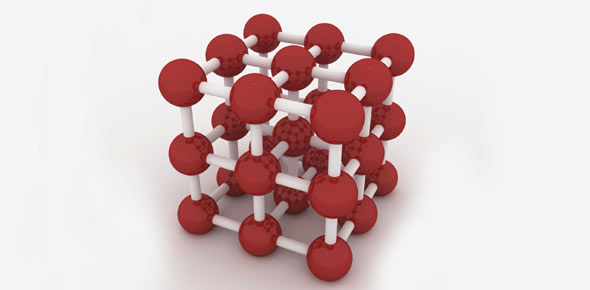# Atoms And Molecules Quiz Questions

15 Questions | Attempts: 178
ShareSettingsHi students. . . . These is a free online test submitted by CHEMISTRY AT 9. . . . . . . BEST OF LUCK

• 1.
If 92.6g of mercury reacts with 7.4g of oxygen then what will be the mass of mercuric oxide?
• A.

100g

• B.

92.4g

• C.

7.4g

• D.

7g

• 2.
If 8.4g of magnesium carbonate on heating gave 4.4g of carbon dioxide and 4.0g of magnesium oxide. These agreement are in agreement with______________
• A.

Law of constant proportion

• B.

Law of conservation of mass

• C.

Law of multiple proportion

• D.

Gay lussac's law

• 3.
Which of the following is one of the drawback of dalton's atomic theory?
• A.

Matter is made up of atoms

• B.

Atoms are indivisible

• C.

Atom is neither created nor destroyed

• D.

An element consists of only one type of atoms

• 4.
The molecular mass of ozone is
• A.

32 u

• B.

16 u

• C.

54 u

• D.

48 u

• 5.
What is the symbol of sodium and copper?
• A.

N and C respectively

• B.

S and C respectively

• C.

Na and Cu respectively

• D.

Na and Co respectively

• 6.
The number of atoms present in a molecule of a substance is
• A.

Molecularity

• B.

Atomicity

• C.

Valency

• D.

Reactivity

• 7.
What is the valency of cabon in carbon dioxide
• A.

2

• B.

1

• C.

4

• D.

3

• 8.
Atomic weight of nitrogen is 14. It means that
• A.

It is 14 times heavier than hydrogen atom d) None of the above Mass of Nitrogen is 14 gram

• B.

It is 14 times heavier than carbon-12 atom

• C.

It is of is grams

• D.

None of the above

• 9.
Name the scientist who gave the atomic theory of matter
• A.

Albert Einstein

• B.

John Dalton

• C.

• D.

Neils Bohr

• 10.
Magnesium and oxygen combine in the ratio of 3:2 by mass to form magnesium oxide. What mass of oxygen gas would be required to react completely with 24 grams of magnesium?
• A.

16 g b) 45 g c) 12 g d) 19 g 16g

• B.

45 g

• C.

12 g

• D.

19 g

• 11.
The smallest atom of all is _________.
• A.

C

• B.

H

• C.

Cu

• D.

Na

• 12.
The value of Avogadro number is _______________.
• A.

9.022 x 10^23 ( ^ = THESE SYMBOL INDICATES TO THE POWER )

• B.

6.022 X 10^23

• C.

12.44 X 10^23

• D.

3.011 X 10^23

• 13.
1 mole = ___________ mass of element.
• A.

Kilogram

• B.

Metre

• C.

Gram atomic

• D.

None of this these

• 14.
What is the valency of nitrogen in ammonia (NH3 )
• A.

3

• B.

4

• C.

2

• D.

1

• 15.
What is the formula of lithium nitride
• A.

LiN

• B.

(LI2)3 N

• C.

Li2N

• D.

Li3N

## Related TopicsBack to top
×

Wait!
Here's an interesting quiz for you.